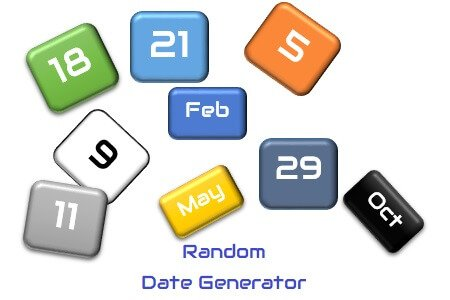# Random Date Generation in LoadRunner## Problem:

How to simulate random date generation scenario in LoadRunner?

## Explanation:

A webpage with the date field(s) requires current, past or future date inputs. The Date/Time parameter can easily generate value in the required date format and by using an offset, LoadRunner can generate past and future dates too. But in the case of date randomization, the value generated by ‘Date/Time Parameter’ does not fulfil the purpose because it generates the value in timestamp format (Date + Time) and if the only date format is selected then the same value (same date) will be generated every time.

In performance testing, the script requires a random date value to pass in the past or future date fields. Some scenarios are like to generate a report between two dates, to book a ticket for a bus etc. These scenarios have one to two date fields and require a date input. So, how to generate a random date in LoadRunner?

``````Action()
{
int randNumber;
randNumber = rand()%60 + 1;  //Generate Random Number 1 to 60
lr_output_message("Random Number is %d", randNumber);
lr_save_datetime("%d/%m/%Y", DATE_NOW + ONE_DAY*randNumber, "randDate");
lr_output_message("Random Date is %s", lr_eval_string("{randDate}"));
return 0;
}

Output:
Starting action Action.
Action.c(5): Random Number is 27
Action.c(7): Random Date is 19/02/2019
Ending action Action.``````

## How does it work?

1. The rand() function generates a random number in the range 0 to 59.
2. The offset 1 increases the range of generated value by 1. Thus the range of random number becomes 1 to 60.
3. The argument DATE_NOW stores the current date.
4. ONE_DAY*randNumber converts the random number into date format.
5. Random Date is added into the current date. (DATE_NOW + ONE_DAY*randNumber)
6. The lr_save_datetime method saves the random date in the randDate variable.

You may be interested:

### 2 Responses

1.M E Vybhav says:

Hi PerfMatrix, can you please explain me the working of this code and as to how and what exactly these functions do. How are the values calculated, could you please tell me.

Thanks and Regards,

M E Vybhav

•PerfMatrix says:

Hi Vybhav,

This is very basic coding logic. Kindly go through any C++ language tutorial and you can understand the code.
In this code, rand() function is generating a value and that is divided by 60. Then add 1 in the mode or remainder of the division. This is because 0 could be one of the modes and we have to generate the value in the range of 1 to 60.

Another time function is used to add the random number in the current date and produce a value in the date format. Rest functions are used to display the output.